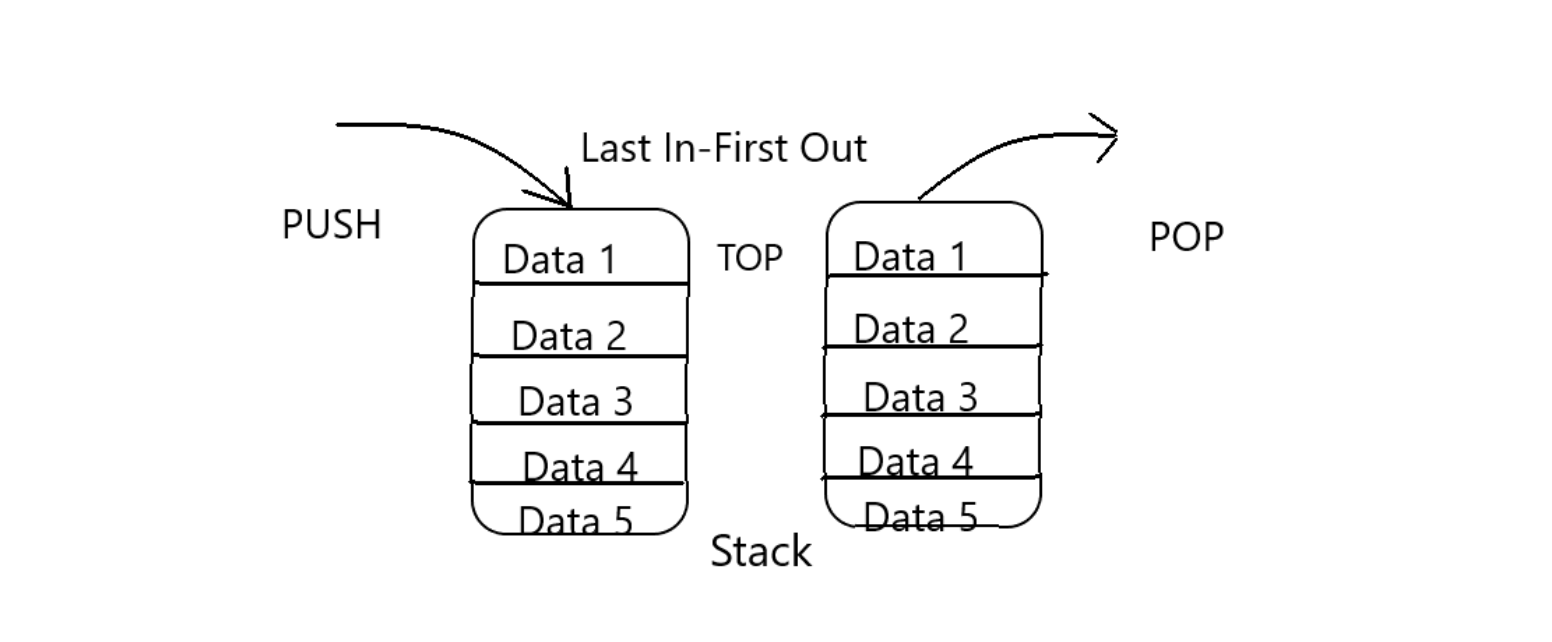The stack is basically a linear data structure which stores its items in a specific order i.e. LIFO (Last In First Out) or FILO (First In Last Out). The element can be added at one end and also can be removed from that end only i.e. only last added element can be removed. The operation of adding an element to the stack is known as push and deletion of the last added element is known as pop.## Implementing Stack in Python:

Unlike Arrays in C language, which is a static linear data structure, Stack is a dynamic linear data structure which allocates the memory whenever needed.

There are some methods associated with stack :

•  push(val) : Insert a value ‘val’ into the stack.
•  pop() : Removes the top most element of stack.
•  top() : Returns the reference of top-most element in the stack.
• size() : Returns the current size of the stack.

The Time Complexity of all the above methods is O(1).

Got familiar about Stack but how can we implement it using Python. Let’s see the various ways to implement it using Python.

• list
• collections.deque
• queue.LifoQueue

## Python Stack using Lists:

The stack can be implemented by using Python Lists i.e. a linear data structure widely used to store any type of variables or other lists sequentially. The list has an in-built method `pop()` which removes the last inserted element, so it can be used to remove an element of the stack in LIFO order. Also, list `append()` method can be used to insert any element to the stack (push()).

``````# Python implementation of a Stack using List

myStack = []

myStack.append(10)
myStack.append(20)
myStack.append(30)
myStack.append(40)

print('The elements in the stack are: ')
print(myStack)

# Note that if the type of Stack is printed
# print(type(myStack))
# it still gives <class 'list'>

# The popped elements can also be tracked
# by printing them

print('\nElements popped are:')
print(myStack.pop())
print(myStack.pop())

print('\nStack after popping out elements')
print(myStack)
``````

Output:

``````The elements in the stack are:
[10, 20, 30, 40]

Elements popped are:
40
30

Stack after popping out elements
[10, 20]
``````

Note that if the `pop()` method is used when the stack is empty then it will give IndexError.

## Python Stack using deque from collections:

Stack can also be implemented by using` deque` class in the collections module. It is preferred over lists widely because of its time complexity is `O(1)` in pop operation as compared to that of lists. Also, `append()` and `pop()` method can be used as lists.

``````# Python implementation of a Stack
# using collections.deque

from collections import deque

myStack = deque()

myStack.append(10)
myStack.append(20)
myStack.append(30)
myStack.append(40)

print('The elements in Stack are:')

# Note that if the type of Stack is printed
# print(type(myStack))
# it gives <class 'collections.deque'>

print(myStack)

print('\nElements popped out from the stack are:')
print(myStack.pop())
print(myStack.pop())

print('\nStack after popping out elements')
print(myStack)``````

Output:

``````The elements in Stack are:
deque([10, 20, 30, 40])

Elements popped out from the stack are:
40
30

Stack after popping out elements
deque([10, 20])
``````

Note that if `pop()` method is used when the stack is empty then it will give `IndexError`.

Now, Let’s see another way to implement a stack. i.e.

## Python Stack using LifoQueue from the queue:

There is a` LifoQueue` class in queue module which is specifically designed to implement a stack in Python. As we have stack STL (Standard Template Library) in C++ and its methods push() and pop(), Here we have put() and get() in-built methods available in the queue module for adding and deleting an element in LIFO order respectively.

Before the implementation, some of the in-built methods of queue module must be covered.

• maxsize: It is the number of elements that can be stored in LifoQueue. (Note that by default maxsize = 0 is initialized if we do not pass maxsize)
• empty() : returns a boolean value whether the LifoQueue is empty.
• full() : returns a boolean value whether the LifoQueue is full.
• qsize() : returns an integer i.e. current size of LifoQueue.
• get() : pop last inserted element and returns the popped value of LifoQueue
• put(val) : inserts an element “val” into the LifoQueue.
``````# Python program to
# Python implementation of a myStack
# using queue.LifoQueue

from queue import LifoQueue

maxm_size = 4
myStack = LifoQueue(maxsize = maxm_size)

# If we print size of LifoQueue it will give 0
print(myStack.qsize())

if myStack.empty():
print('Stack is Empty')

myStack.put(10)
myStack.put(20)
myStack.put(30)
myStack.put(40)

if myStack.full():
print('Stack is Full')

print('\nElements popped out from the stack are:')
print(myStack.get())
print(myStack.get())

print('\nStack after popping out elements')

# Also Note that if we use print(myStack)
# then it will give the object reference
# like <queue.LifoQueue object at 0x7fc5662d3748>
# print(myStack)

# myStack.queue returns an iterable list
# of the current elements in LifoQueue
print(myStack.queue)
``````

Output:

``````0
Stack is Empty
Stack is Full

Elements popped out from the stack are:
40
30

Stack after popping out elements
[10, 20]
``````

## References:

Happy Learning 🙂